## Electronic T/R Switching and the Ameritron QSK-5 by Greg Latta AA8V How PIN Diodes and PIN Diode Switches Work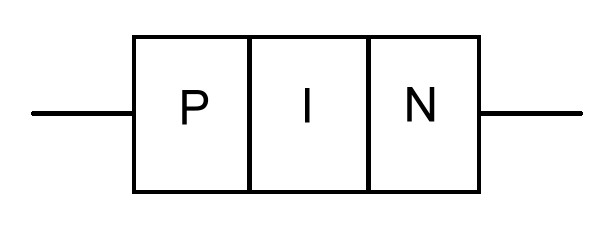Structure of a PIN Diode

 Main Page Handshake Circuit How PIN Diodes and PIN Diode Switches Work QSK Indicator Modification Building an Electronic Transmit/Receive Switch Using PIN Diode Switches Hot Switching PIN Switch Driver - Turning A PIN Diode Switch Off and On Circuit Board And Parts Identification T/R Input and Timing Circuits Schematic Diagram and Circuit Descriptions

Safety Note: Working on or testing the QSK-5 and associated circuitry may involve operating the amplifier containing the QSK-5 with the cover off. This is extremely dangerous since very high voltages are present when the amplifier is turned on, sometimes in close proximity to the QSK-5. If at all possible, do all work with the amplifier off and unplugged. The operator assumes all risk and liability in such matters! Never operate the amplifier with the cover off unless you are experienced with working around very high voltages!

What Is A PIN Diode:
Conventional PN junction diodes such as the 1N4005 diode often used in power supplies consist of a region of positively doped (P) and negatively doped (N) silicon bonded together as shown below: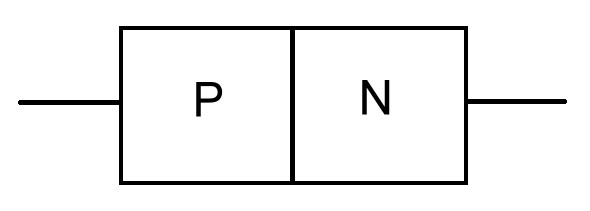Structure of a PN Diode

A PIN diode is a junction diode with a region of undoped (intrinsic=I) silicon layered between the conventional positively doped (P) and negatively doped (N) regions, as can be seen in the diagram below:Structure of a PIN Diode

The addition of the intrinsic region greatly alters the behavior of the diode at radio frequencies by lowering the capacitance between the P and N regions and raising the time it takes for the diode to switch between the conducting and non-conducting modes.

How is the behavior of a PIN diode different from that of a conventional PN diode?
At lower frequencies, such as power line frequencies and audio frequencies, a PIN diode behaves the same as regular diode. It passes current in only one direction. However, at radio frequencies the PIN diode acts very differently from a conventional diode.

At radio frequencies, a PIN diode acts as either a small valued capacitor or as a variable resistor, depending on the DC bias applied to the diode. If the diode is reverse biased at DC, at RF it behaves as a very small value capacitor with a capacitance of approximately 1 pf. Though RF can get through a 1 pf capacitor, the amount that gets through is quite small. If the leakage through 1 pf is a problem, several diodes can be strung in series, reducing the capacitance and thus the residual leakage. Thus, for RF, the PIN diode acts as an open switch if it is reverse biased with DC. Even though the alternating RF current superimposed on the DC may occasionally forward bias the diode, the slow response time caused by the extra "I" layer prevents it from turning on.

If the PIN diode is forward biased at DC, for RF it behaves like a resistor. The RF resistance is inversely proportional to the forward DC bias current. The resistance might be as high at 10,000 ohms for very small forward currents, and as low as 0.1 ohm if the forward DC bias current is 100 mA. Each type of diode has a different resistance vs. forward bias characteristic. If the forward current through the diode is near the maximum the diode is designed for, it typically has an RF resistance of only 0.1 ohm, and thus acts as a closed switch. Thus, for RF, the PIN diode acts as a closed switch if it is forward biased with the near maximum DC bias current. Even though the alternating RF current superimposed on the DC may occasionally reverse bias the diode, the slow response time caused by the extra "I" layer prevents it from turning off.

If we can apply both DC and RF to a PIN diode at the same time, we can use the PIN diode as an RF switch. Reverse biasing the diode at DC turns the switch off, and forward biasing the diode at DC turns on the switch. The resulting "solid state switch" has a switching time much faster than any mechanical switch or relay.

How do you apply both DC and RF to the diode at the same time?
This isn't at hard as it might seem. Since capacitors block DC while passing RF, and RF chokes block RF while passing DC, a combination of the two ought to do the trick. Take a look at the diagram below, which is a schematic of a simple single pole/single throw RF switch using a PIN diode: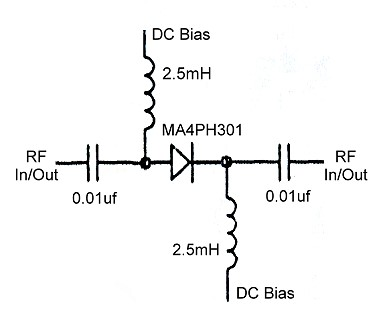Applying both RF and DC Bias at the Same Time

As can be seen in the diagram, the RF is fed into and out of the diode through 0.01uf blocking capacitors. These allow the RF to pass through to the diode while preventing the DC bias from getting into the RF circuits. Meanwhile, the DC bias is applied to the diode through a pair of 2.5mH RF chokes. These allow the DC to reach the diode while preventing any RF from getting into the bias circuit.

In the diagram above, if about 200V DC is connected to the DC bias terminals (negative connection going to the top) the diode will be reversed biased and the switch will be shut off. Very little current is needed from the 200V supply, since the diode is reverse biased.

If about 50mA from a much lower voltage supply is allowed to flow through the diode in the forward direction (positive connection going to the top), then the diode will be forward biased and the switch will be turned on.

By the way, don't let the diode arrow mislead you. As far as the RF is concerned, it isn't a diode, but a switch/resistor, and the RF is perfectly happy to go through the diode either way!

The switch above can only handle low power and is designed to be used as a switch in a receiving or QRP circuit.

What if I need better isolation when the switch is off?
If the leakage through one diode when the switch is turned off is too much, then the first thing you must do is to be sure that you are applying the largest reverse bias possible. Raising the reverse bias lowers the capacitance of the diode, reducing the leakage. If raising the bias to the maximum doesn't provide enough isolation, then you need to use more than one diode in series, as shown below: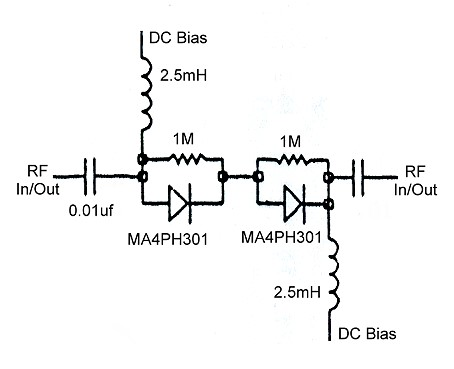Putting Diodes in Series to Provide Better Isolation

This is the same circuit as before, but two diodes are now in series, with a 1Mohm equalizing resistor connected across each to make sure that the reverse bias is split equally between the diodes. Note that you must now double the reverse bias voltage so that each diode receives the maximum it can withstand. The effective capacitance of the two diodes in series is half the capacitance of a single diode. You can use as many diodes as needed. The Ameritron QSK-5 uses a total of eight diodes in series (in a slightly different configuration) in the receive switch to get enough isolation between the input and output of the amplifier.

What if I need more power handling capability?
If the switch needs to handle more power, one option is to use a larger PIN diode. However, there is practical limit to diode size, and if the power cannot be handled by a single diode, then it is possible to place diodes in parallel to handle higher power. Note that the DC bias to the diodes should be applied in series to assure that the forward bias is the same in each. This also reduces the current demand on the bias power supply. However, the diodes must be connected in parallel for RF. See the diagram below: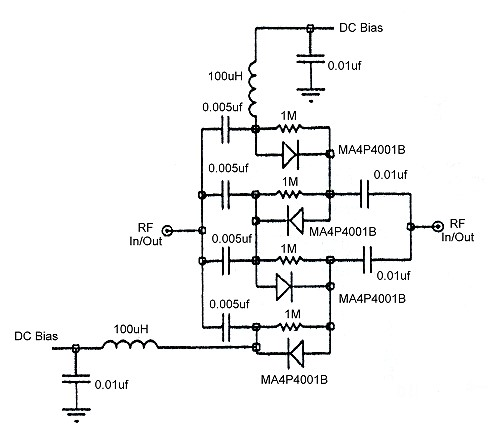Putting Diodes in Parallel for RF but in Series for DC to Increase Power Handling

In the circuit above, which is used in the Ameritron QSK-5, the forward DC bias current flows from top to bottom in series through the diodes. This guarantees that the same bias current is flowing through each diode so that the RF resistance is the same for each. The diodes are, however, connected in parallel for RF, and the values of the coupling capacitors are chosen so that the RF current is split equally among the four diodes. Recall that RF doesn't care which direction it flows through the diodes, since at RF the diodes act like switches/resistors. In this case the RF resistance of each diode is roughly 0.1ohm to 0.2ohm, so that the effective parallel RF resistance is 0.025ohm to 0.05ohm, which is essentially a short circuit. When the diodes are reverse biased, the 1Mohm equalizing resisters assure that the reverse bias is split equally between all of the diodes. In the circuit above the reverse bias should be four times that needed by a single diode to insure that the diodes are fully cut off.

The RF chokes used in this circuit are 100uH instead of 2.5mH, as used in the other switches shown above. This is because the 2.5mH chokes can't handle the larger forward bias current of the larger diodes. To make sure that no residual RF gets through the chokes into the bias supply, the end of each choke is bypassed to ground with a 0.01uf capacitor.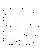If you have any questions or comments, you can send E-Mail to Dr. Greg Latta at glatta@frostburg.edu